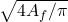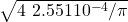## when a temparature of a coin is 75°C, the coin’s diameter increases. if the original diameter of a coin is 1.8*10^-2 m and its coefficient o

Question

when a temparature of a coin is 75°C, the coin’s diameter increases. if the original diameter of a coin is 1.8*10^-2 m and its coefficient of linear expansion is 1.7*10^5/°C, what is the change in coins diameter?​

in progress 0
3 months 2021-07-19T16:37:15+00:00 1 Answers 2 views 0

ΔD = 2.29 10⁻⁵ m

Explanation:

This is a problem of thermal expansion, if the temperature changes are not very large we can use the relation

ΔA = 2α A ΔT

the area is

A = π r² = π D² / 4

we substitute

ΔA = 2α π D² ΔT/4

as they do not indicate the initial temperature, we assume that ΔT = 75ºC

α = 1.7 10⁻⁵ ºC⁻¹

we calculate

ΔA = 2 1.7 10⁻⁵ pi (1.8 10⁻²) ² 75/4

ΔA = 6.49 10⁻⁷ m²

by definition

ΔA = A_f- A₀

A_f = ΔA + A₀

A_f = 6.49 10⁻⁷ + π (1.8 10⁻²)² / 4

A_f = 6.49 10⁻⁷ + 2.544 10⁻⁴

A_f = 2,551 10⁻⁴ m²

the area is

A_f = π D_f² / 4

A_f =D_f =D_f = 1.80229 10⁻² m

the change in diameter is

ΔD = D_f – D₀

ΔD = (1.80229 – 1.8) 10⁻² m

ΔD = 0.00229 10⁻² m

ΔD = 2.29 10⁻⁵ m